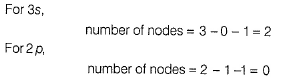Courses

# Retro (Past 13 Years) IIT - JEE Advanced (Structure Of Atom)

## 11 Questions MCQ Test Chemistry for JEE Advanced | Retro (Past 13 Years) IIT - JEE Advanced (Structure Of Atom)

Description
This mock test of Retro (Past 13 Years) IIT - JEE Advanced (Structure Of Atom) for JEE helps you for every JEE entrance exam. This contains 11 Multiple Choice Questions for JEE Retro (Past 13 Years) IIT - JEE Advanced (Structure Of Atom) (mcq) to study with solutions a complete question bank. The solved questions answers in this Retro (Past 13 Years) IIT - JEE Advanced (Structure Of Atom) quiz give you a good mix of easy questions and tough questions. JEE students definitely take this Retro (Past 13 Years) IIT - JEE Advanced (Structure Of Atom) exercise for a better result in the exam. You can find other Retro (Past 13 Years) IIT - JEE Advanced (Structure Of Atom) extra questions, long questions & short questions for JEE on EduRev as well by searching above.
*Answer can only contain numeric values
QUESTION: 1

### Retro IIT-JEE Advanced  (Compilation of Last 10 Years  Questions) IIT JEE (Main & Advanced) past year questions with solutions & answer key Retro IIT JEE (Main and Advanced) have questions from previous/past (10 to 13 years) papers of IIT JEE (Main and Advanced) with solutions, The following set of questions contain previous/past (10 to 13 years) questions of IIT JEE (Main and Advanced) of Chapter "Structure of Atom" with solutions  Q. In an atom, the total number of electrons having quantum numbers n = 4,| ml | = 1 and ms = - 1/2 is (2014 Adv., Integer Type)

Solution:

|ml| = 1thus, magnetic quantum number = + 1 and -1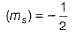n = 4 can have suborbits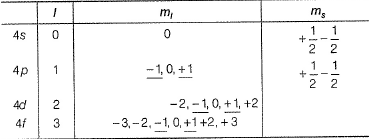Thus underlined electrons with (m, = - 1, + 1) and spin - (1/2) are six.

*Answer can only contain numeric values
QUESTION: 2

### The atomic masses of He and Ne are 4 and 20 amu, respectively. The value of the de-Broglie wavelength of He gas at -73°C is "M" times that of the de-Broglie wavelength of Ne at 727°C. M is (2013 Adv., Integer Type)

Solution: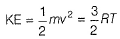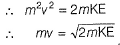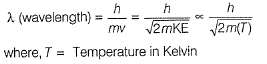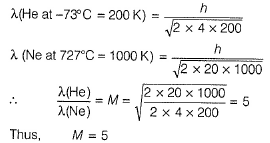QUESTION: 3

### The kinetic energy of an electron in the second Bohr orbit of a hydrogen atom is [a0 is Bohr radius] (2012, Single Option Correct Type)

Solution:

According to Bohr's model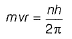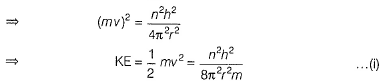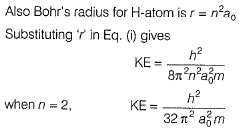*Answer can only contain numeric values
QUESTION: 4

The maximum number of electrons that can have principal quantum number, n = 3 and spin quantum number, ms = - 1 / 2 , is

(2011, Integer Type)

Solution:

When n = 3, l = 0,1,2, i.e. there are 3s, 3p and 3d orbitals. If all these orbitals are completely occupied as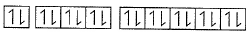Total 18 electrons, 9 electrons with s =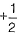and 9 with s =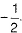Alternatively
In any nth orbit, there can be a maximum of 2n2 electrons. Hence, when n = 3, number of maximum electrons = 18. Out of these 18 electrons, 9 can have spin =and remaining nine with spin =*Answer can only contain numeric values
QUESTION: 5

The work function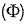of some metals is listed below. The number of metals which will show photoelectric effect when light of 300 nm wavelength falls on the metal is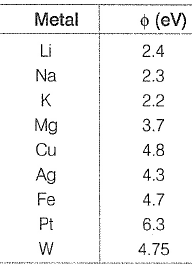(2011, integer Type)

Solution: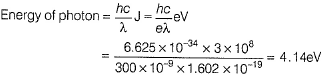For photoelectric effect to occur, energy of incident photons must be greater than work function of metal. Hence, only Li, Na, K and Mg have work functions less than 4.14V.

QUESTION: 6

Direction (Q. Nos. 6-8) This section contains a paragraph, wach describing  theory, experiments, data etc. three Questions related to paragraph have been  given.Each question have only one correct answer among the four given  ptions  (a),(b),(c),(d)

The hydrogen-like species Li2+ is in a spherically symmetric state S1, with one radial node. Upon absorbing light the ion undergoes transition to a state S2. The state S2 has one radial node and its energy is equal to the ground state energy of the hydrogen atom.

Q. The stateS1 is

Solution:

S1 is spherically symmetrical state, i.e. it corre spo nd to a s-orbital. Also it has one radial node.
Number of radial nodes = n - I - I
n - 0 - 1 = 1
n = 2, i.e. S1 = 2s orbital.

QUESTION: 7

The hydrogen-like species Li2+ is in a spherically symmetric state S1, with one radial node. Upon absorbing light the ion undergoes transition to a state S2. The state S2 has one radial node and its energy is equal to the ground state energy of the hydrogen atom.

Q. Energy of the state S1 in units of the hydrogen atom ground state energy is

Solution:

Ground state energy of electron in H-atom (EH)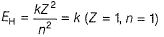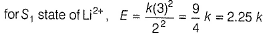QUESTION: 8

The hydrogen-like species Li2+ is in a spherically symmetric state S1, with one radial node. Upon absorbing light the ion undergoes transition to a state S2. The state S2 has one radial node and its energy is equal to the ground state energy of the hydrogen atom.

Q. The orbtial angular momentum quantum number of the state S2 is

Solution: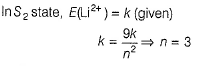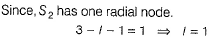QUESTION: 9

Match the entries in Column I with the correctly related quantum number(s) in Column II.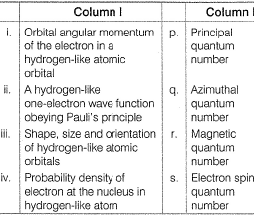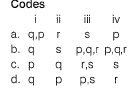Solution: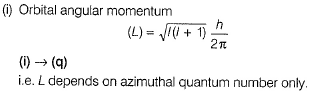(ii) To describe a one electron wave function, three quantum numbers n, I and m are needed. Further to abide by Pauli exclusion principle, spin quantum number (s) is also needed,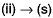(iii) For shape, size and orientation, only n, I and m are needed.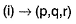(iv) Probability density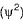can be determined if n, I and m are known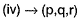QUESTION: 10

According to Bohr’s theory
En = Total energy, Kn = Kinetic energy
Vn = Potential energy, rn = Radius of nth orbit

Match the following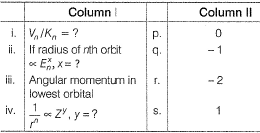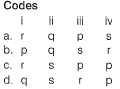Solution: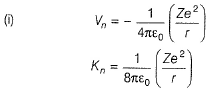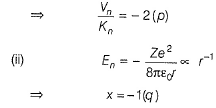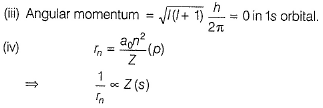QUESTION: 11

The number of radial nodes in 3s and 2p respectively are

(2005, Single Option Correct Type)

Solution:

The number of radial nodes is given by expression (n - l - 1).